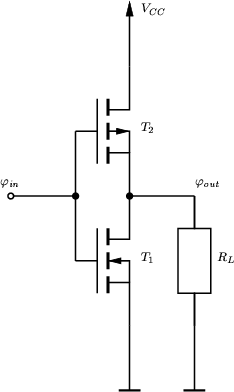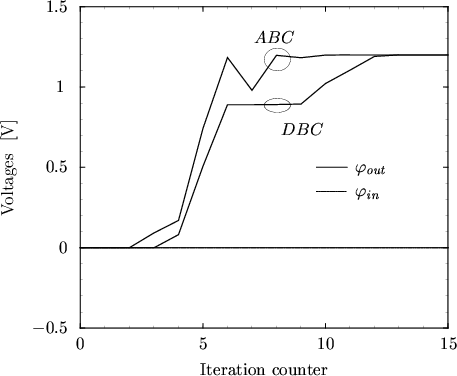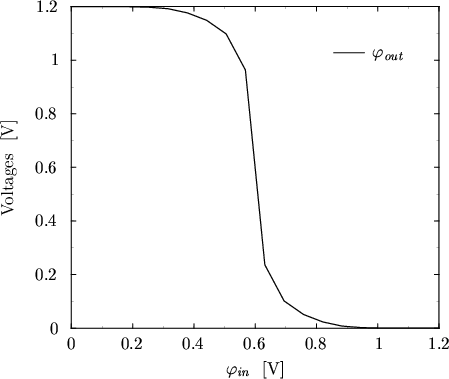Next: 7. Simulation Results Up: 6.3 Examples Previous: 6.3.2 CML Inverter

## 6.3.3 CMOS Inverter

The schematic of a CMOS inverter is shown in Fig. 6.15. The evolution of the node voltages during DC operating point calculation is shown in Fig. 6.16. Best results were obtained with= 1. With ABC 21 iterations were needed whereas with DBC 25 iterations were needed.

For ABC one gets KA = 21/24 = 0.875 and for DBC KD = 25/27 = 0.926, both values being even better than the ideal'' simulation. Again, as for the CML inverter, each point of the DC transfer characteristic required the same number of iterations for both boundary conditions (7-17).

For this simple circuit convergence can be obtained without any GS at a convergence rate similar to k0.Tibor Grasser
1999-05-31